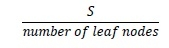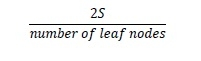# Assign weights to edges such that longest path in terms of weights is minimized in C++

C++Server Side ProgrammingProgramming

Here we will see one problem, in this problem one edge of a tree and a sum S is given. The task is to assign weights to all other weights, such that longest path in terms of weight is minimized. The sum of weights assigned to it is same as ‘S’.

The approach is simple. The property of a tree that a path can have a maximum of two leaf nodes in it. That will be used to get the solution. So if we assign weights only to the edges connecting the leaf nodes, and assign other edges to 0. Then every edge which is connecting to the leaf nodes will be assigned asSince a path can contain a maximum of two leaf nodes, hence the longest path will be## Example

#include<iostream>
#include<vector>
using namespace std;
void insertEdge(int u, int v, vector<int> adj[]) {
}
long double pathLength(vector<int> adj[], int sum, int n) {
int count = 0;
for (int i = 1; i <= n; i++) {
count++;
}
long double ans = 2.0 * (long double)(sum / (long double)(count));
return ans;
}
int main() {
int n = 6;
}
0.5